Finding Inverse

Chapter 1 Class 12 Relation and Functions
Concept wise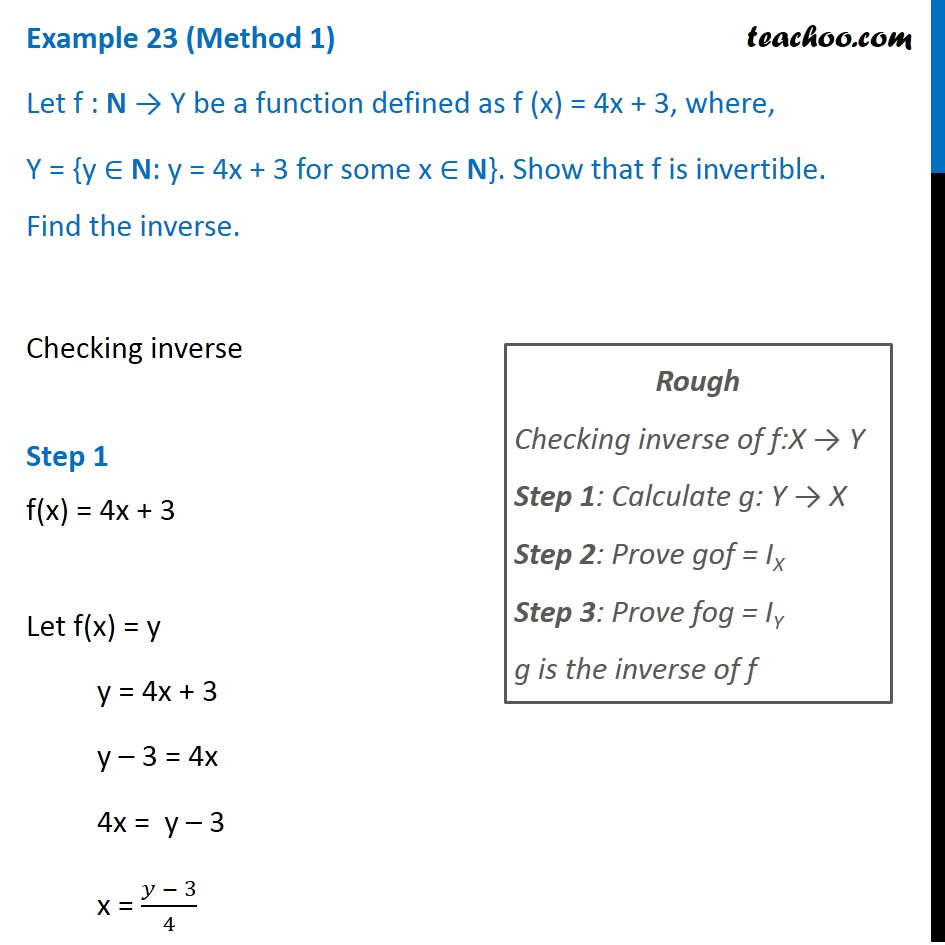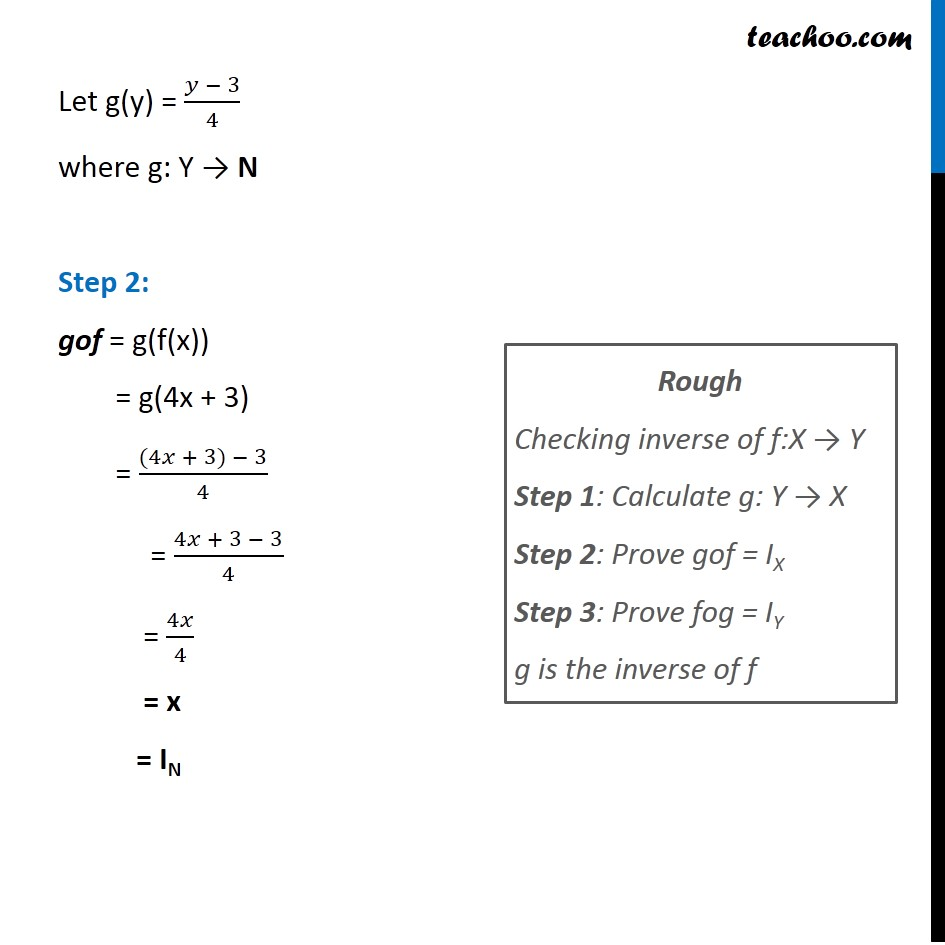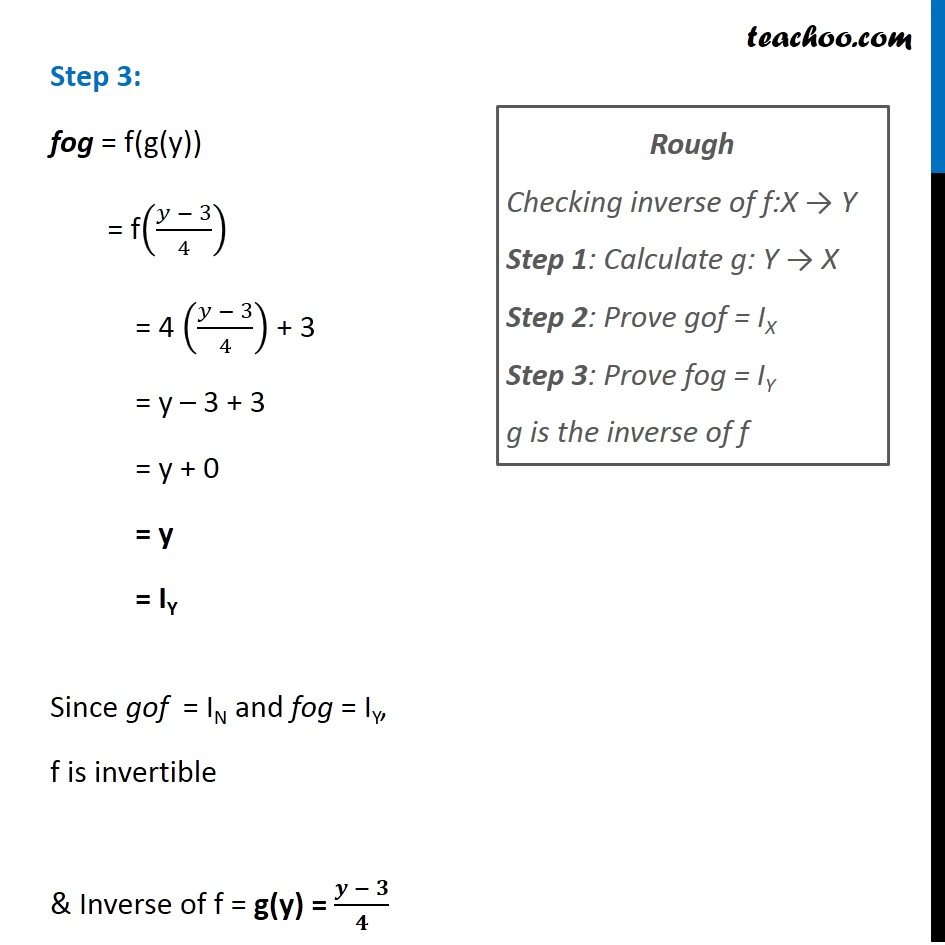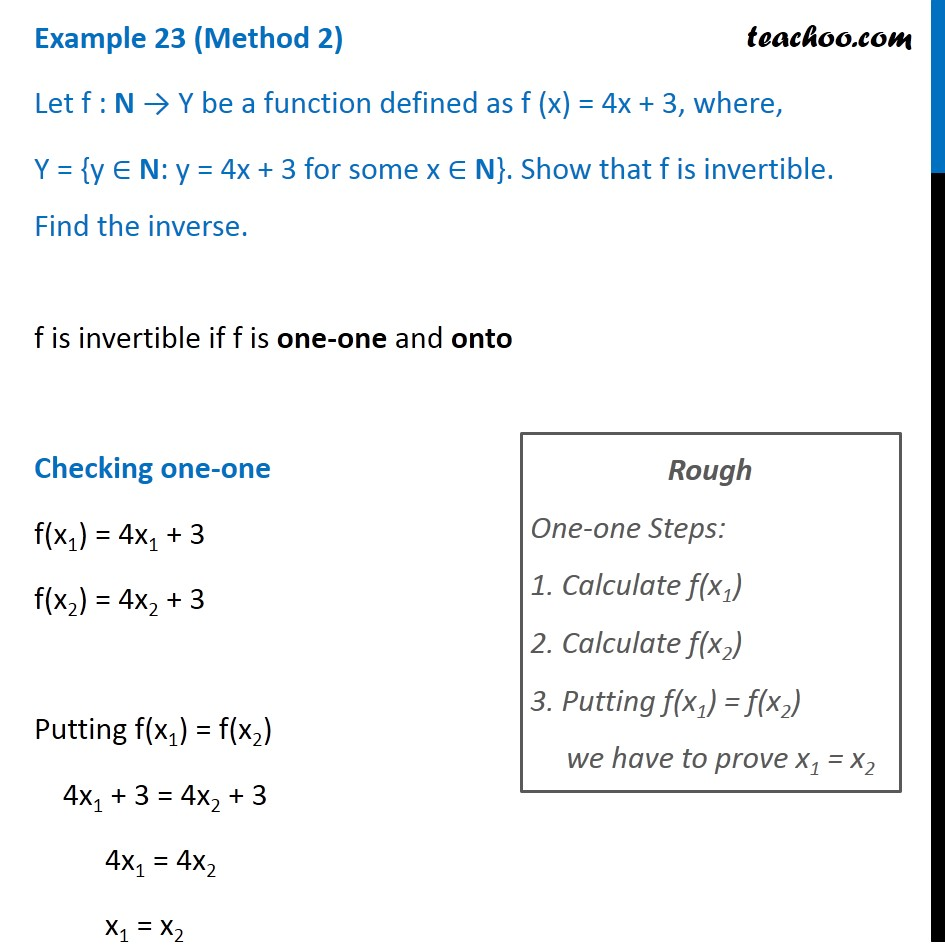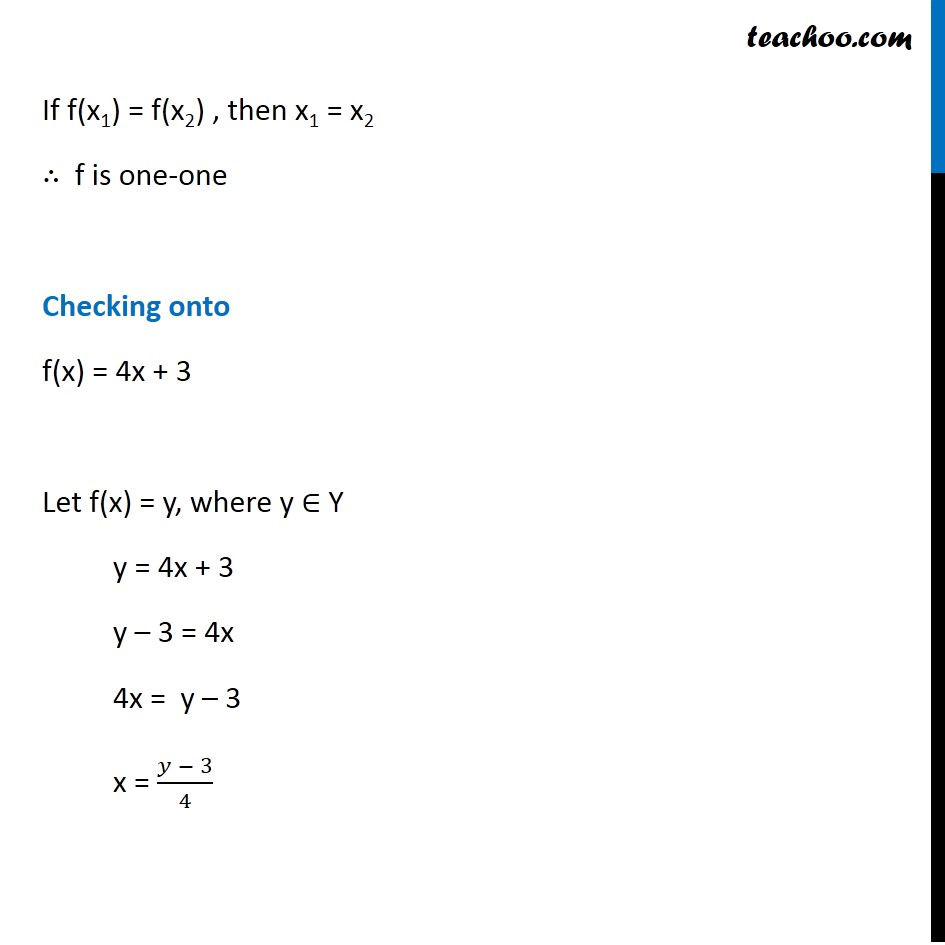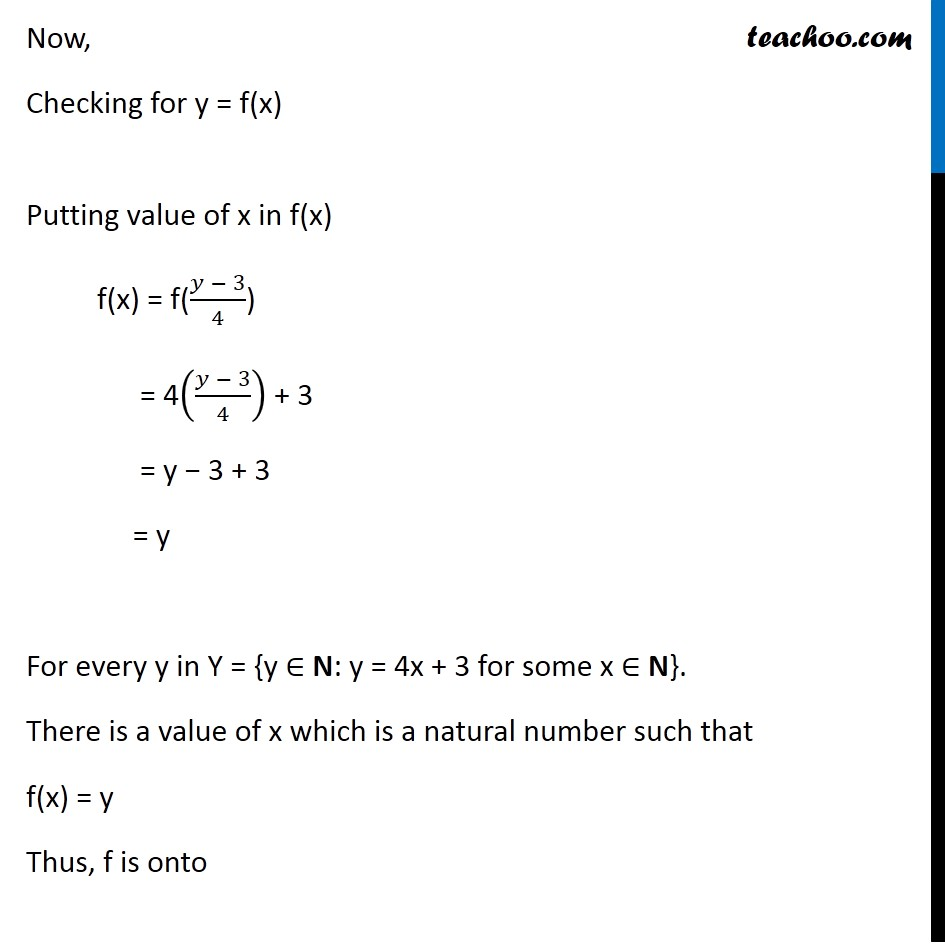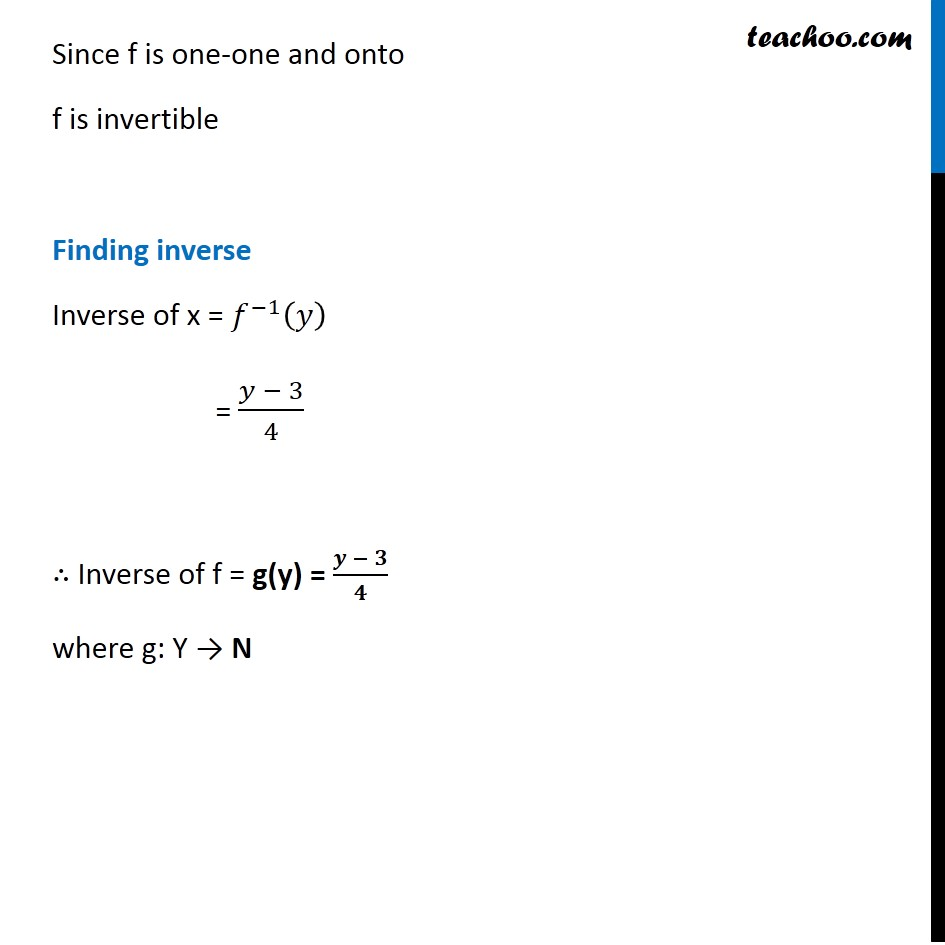Learn in your speed, with individual attention - Teachoo Maths 1-on-1 Class

### Transcript

Example 17 (Method 1) Let f : N → Y be a function defined as f (x) = 4x + 3, where, Y = {y ∈ N: y = 4x + 3 for some x ∈ N}. Show that f is invertible. Find the inverse. Checking inverse Step 1 f(x) = 4x + 3 Let f(x) = y y = 4x + 3 y – 3 = 4x 4x = y – 3 x = (𝑦 − 3)/4 Rough Checking inverse of f:X → Y Step 1: Calculate g: Y → X Step 2: Prove gof = IX Step 3: Prove fog = IY g is the inverse of f Let g(y) = (𝑦 − 3)/4 where g: Y → N Step 2: gof = g(f(x)) = g(4x + 3) = ((4𝑥 + 3) − 3)/4 = (4𝑥 + 3 − 3)/4 = 4𝑥/4 = x = IN Rough Checking inverse of f:X → Y Step 1: Calculate g: Y → X Step 2: Prove gof = IX Step 3: Prove fog = IY g is the inverse of f Step 3: fog = f(g(y)) = f((𝑦 − 3)/4) = 4 ((𝑦 − 3)/4) + 3 = y – 3 + 3 = y + 0 = y = IY Since gof = IN and fog = IY, f is invertible & Inverse of f = g(y) = (𝒚 − 𝟑)/𝟒 Rough Checking inverse of f:X → Y Step 1: Calculate g: Y → X Step 2: Prove gof = IX Step 3: Prove fog = IY g is the inverse of f Example 17 (Method 2) Let f : N → Y be a function defined as f (x) = 4x + 3, where, Y = {y ∈ N: y = 4x + 3 for some x ∈ N}. Show that f is invertible. Find the inverse. f is invertible if f is one-one and onto Checking one-one f(x1) = 4x1 + 3 f(x2) = 4x2 + 3 Putting f(x1) = f(x2) 4x1 + 3 = 4x2 + 3 4x1 = 4x2 x1 = x2 Rough One-one Steps: 1. Calculate f(x1) 2. Calculate f(x2) 3. Putting f(x1) = f(x2) we have to prove x1 = x2 If f(x1) = f(x2) , then x1 = x2 ∴ f is one-one Checking onto f(x) = 4x + 3 Let f(x) = y, where y ∈ Y y = 4x + 3 y – 3 = 4x 4x = y – 3 x = (𝑦 − 3)/4 Now, Checking for y = f(x) Putting value of x in f(x) f(x) = f((𝑦 − 3)/4) = 4((𝑦 − 3)/4) + 3 = y − 3 + 3 = y For every y in Y = {y ∈ N: y = 4x + 3 for some x ∈ N}. There is a value of x which is a natural number such that f(x) = y Thus, f is onto Since f is one-one and onto f is invertible Finding inverse Inverse of x = 𝑓^(−1) (𝑦) = (𝑦 − 3)/4 ∴ Inverse of f = g(y) = (𝒚 − 𝟑)/𝟒 where g: Y → N